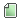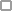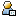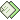Pagini:    În josAjutor Subiect: Assignments - Programming Methodology - Stanford  (Citit de 21691 ori) 0 Utilizatori şi 1 Vizitator pe acest subiect.
siliconvalleyguy
Strain

Karma: -2Deconectat

Mesaje: 6« : Septembrie 21, 2011, 08:00:46 »

Am gasit de curand, pe internet, Cursul "Programming Methodology" al celor de la Stanford si cred ca este o referinta buna pentru oricine nu are experienta ca programator si vrea sa invete, de acasa, programare. Pe http://www.stanford.edu/class/cs106a/ se pot gasi toate materialele de care este nevoie pentru a invata Java, LIVE - la inceput cu ajutorul lui Karel - The Robot.
Pentru cine prefera varianta on-line a cursului: http://www.siliconvalleyplayer.com/p/stanford.html

/*
* Daca se pricepe cineva la PDF-uri ar putea completa aceasta sectiune cu temele din Assignments - eu nu stiu cum sa introduc imaginile.
*/
 « Ultima modificare: Octombrie 19, 2011, 11:57:13 de către Craciun Bogdan-George »Memorat
siliconvalleyguy
Strain

Karma: -2Deconectat

Mesaje: 6« Răspunde #1 : Octombrie 19, 2011, 11:23:01 »

The Art and Science of Java - Eric S. Roberts - Stanford University - Stanford, California - January 2006

Chapter 2
Programming by Example

Programming exercises
1. Type in the HelloProgram.java program exactly as it appears in this chapter and
name as a signature to the lower right corner.
2. The following program was written without comments or instructions to the user,
except for a couple of input prompts:
import acm.program.*;
public class MyProgram extends ConsoleProgram {
public void run() {
double b = readDouble("Enter b: ");
double h = readDouble("Enter h: ");
double a = (b * h) / 2;
println("a = " + a);
}
}
Read through the program and figure out what it is doing. What result is it
calculating? Rewrite this program so it is easier to understand, both for the user and
for the programmer who must modify the program in the future.
3. Extend the Add2Integers program given in Figure 2-2 so that it adds three numbers
4. Write a GraphicsProgram subclass that generates a picture of a
snowperson.Memorat
siliconvalleyguy
Strain

Karma: -2Deconectat

Mesaje: 6« Răspunde #2 : Octombrie 19, 2011, 11:31:01 »

Chapter 3
Expressions

1. Extend the InchesToCentimeters program given in Figure 3-2 so that it reads in
two input values: the number of feet, followed on a separate line by the number of
inches.
2. Write a program that reads in two numbers: an account balance and an annual
interest rate expressed as a percentage. Your program should then display the new
balance after a year. There are no deposits or withdrawals—just the interest payment.
3. Extend the program you wrote in Exercise 2 so that it also displays the balance after
two years have elapsed, Note that the interest used in this example is compounded annually, which means the
interest from the first year is added back to the bank balance and is therefore itself
subject to interest in the second year. In the first year, the \$6,000 earns 4.25%
interest, or \$255. In the second year, the account earns 4.25% interest on the entire
\$6,255.
4. Write a program that asks the user for the radius of a circle and then computes the
area of that circle (A) using the formula
A = π * r * r
5. Write a program that reads in a temperature in degrees Fahrenheit and returns the
corresponding temperature in degrees Celsius.The conversion formula is
C = (5/9) * (F – 32)
6. In Norton Juster’s children’s story The Phantom Tollbooth, the Mathemagician gives
Milo the following problem to solve:
4 + 9 - 2 * 16 + 1 / 3 * 6 - 67 + 8 * 2 - 3 + 26 - 1 / 34 + 3 / 7 + 2 - 5
According to Milo’s calculations, which are corroborated by the Mathemagician, this
expression “all works out to zero.” If you do the calculation, however, the
expression comes out to zero only if you start at the beginning and apply all the
operators in strict left-to-right order. What would the answer be if the
Mathemagician’s expression were evaluated using Java’s precedence rules? Write a
7. Write a program that converts a metric weight in kilograms to the corresponding
English weight in pounds and ounces. The conversion factors you need are
1 kilogram = 2.2 pounds
1 pound = 16 ounces
8. Write a program that computes the average of four integers.
9. There’s an old nursery rhyme that goes like this:
As I was going to St. Ives,
I met a man with seven wives,
Kits, cats, sacks, and wives,
How many were going to St. Ives?
The last line turns out to be a trick question: only the speaker is going to St. Ives;
everyone else is presumably heading in the opposite direction. Suppose, however,
that you want to find out how many representatives of the assembled multitude—
kits, cats, sacks, and wives—were coming from St. Ives. Write a Java program to
calculate and display this result. Try to make your program follow the structure of
the problem so that anyone reading your program would understand what value it is
calculating.Memorat
siliconvalleyguy
Strain

Karma: -2Deconectat

Mesaje: 6« Răspunde #3 : Octombrie 19, 2011, 11:45:53 »

Chapter 4
Statement Forms

Programming exercises
1. As a way to pass the time on long bus trips, young people growing up in the United
States have been known to sing the following rather repetitive song:
99 bottles of beer on the wall.
99 bottles of beer.
You take one down, pass it around.
98 bottles of beer on the wall.
98 bottles of beer on the wall. . . .
Anyway, you get the idea. Write a Java program to generate the lyrics to this song.
(Since you probably never actually finished singing it, you should decide how you
want the song to end.) In testing your program, it would make sense to use some
constant other than 99 as the initial number of bottles.
2. While we’re on the subject of silly songs, another old standby is “This Old Man,” for
which the first verse is
This old man, he played 1.
He played knick-knack on my thumb.
This old man came rolling home.
Each subsequent verse is the same, except for the number and the rhyming word at
the end of the second line, which gets replaced as follows:
2—shoe 5—hive 8—pate
3—knee 6—sticks 9—spine
4—door 7—up to heaven 10—shin
Write a program to display all 10 verses of this song.
3. Write a program that reads in a positive integer N and then calculates and displays
the sum of the first N odd integers. For example, if N is 4, your program should
display the value 16, which is 1 + 3 + 5 + 7.
4. Why is everything either at sixes or at sevens?
— Gilbert and Sullivan, H.M.S. Pinafore, 1878
Write a program that displays the integers between 1 and 100 that are divisible by
either 6 or 7.
5. Repeat exercise 4, but this time have your program display only those numbers that
are divisible by 6 or 7 but not both.
6. Using the AddIntegerList program from Figure 4-5 as a model, write a program
called AverageList that reads in a list of integers representing exam scores and then
prints out the average. Because some unfortunate student might actually get a score
of 0, your program should use –1 as the sentinel to mark the end of the input.
7. Rewrite the DigitSum program given in Figure 4-6 so that instead of adding the
digits in the number, it generates the number that has the same digits in the reverse
order.
8. Rewrite the Countdown program given in Figure 4-8 so that it uses a while loop
9. In mathematics, there is a famous sequence of numbers called the Fibonacci
sequence after the thirteenth-century Italian mathematician Leonardo Fibonacci. The
first two terms in this sequence are 0 and 1, and every subsequent term is the sum of
the preceding two. Thus the first several numbers in the Fibonacci sequence are as
follows:
F0 = 0
F1 = 1
F2 = 1 (0 + 1)
F3 = 2 (1 + 1)
F4 = 3 (1 + 2)
F5 = 5 (2 + 3)
F6 = 8 (3 + 5)
Write a program to display the values in this sequence from F0 through F15.
10. Modify the program in the preceding exercise so that instead of specifying the index
of the final term, the program displays those terms in the Fibonacci sequence that are
less than 10,000.Memorat
siliconvalleyguy
Strain

Karma: -2Deconectat

Mesaje: 6« Răspunde #4 : Octombrie 19, 2011, 11:55:47 »

Chapter 5
Methods

1. Write a program that displays the value of the mathematical constant
ƒÓ = (1 + sqrt(5)) / 2
This constant ƒÓ is called the golden ratio. Classical mathematicians believed that this
number represented the most aesthetically pleasing ratio for the dimensions of a
rectangle, but it also turns up in computational mathematics.
2. In high-school algebra, you learned that the standard quadratic equation
ax2 + bx + c = 0
has two solutions given by the formula
x = (-b +/- sqrt (b.2 +/- 4ac)) / 2a
The first solution is obtained by using + in place of +/- the second is obtained by
using - in place of +/-. Write a Java program that accepts values for a, b, and c, and then calculates the two
solutions. If the quantity under the square root sign is negative, the equation has no
real solutions, and your program should display a message to that effect. You may
assume that the value for a is nonzero.
3. The Fibonacci sequence, in which each new term is the sum of the preceding two,
was introduced in Chapter 4, exercise 9. Rewrite the program requested in that
exercise, changing the implementation so that your program calls a method
fibonacci(n) to calculate the nth Fibonacci number. In terms of the number of
mathematical calculations required, is your new implementation more or less
efficient that the one you used in Chapter 4?
4. Write a method raiseIntToPower that takes two integers, n and k, and returns nk.
Use your method to display a table of values of 2k for all values of k from 0 to 10.
5. Write a method raiseRealToPower that takes a floating-point value x and an integer
k and returns xk. Implement your method so that it can correctly calculate the result
when k is negative.
Use your method to display a table of values of ðk for all values of k from –4 to 4.
6. Write a method nDigits(n) that returns the number of digits in the integer n, which
you may assume is positive. Design a main program to test your method. For hints
about how to write this program, you might want to look back at the DigitSum
program that was given in Figure 4-6.
7. Write a predicate method isPerfectSquare(n) that returns true if the integer n is a
perfect square. Remember that the method Math.sqrt returns a double, which is
therefore only an approximation of the actual square root.
8. Write a predicate method askYesNoQuestion(str) that prints out the string str as
a question for the user and then waits for a response. If the user enters the string
"yes", the askYesNoQuestion method should return true; if the user enters "no",
the method should return false. If the user enters anything else, askYesNoQuestion
should remind the user that it is seeking a yes-or-no answer and then repeat the
question.
9. The values of the combinations method used in the text are often displayed in the
form of a triangle using the following arrangement:
C(0,0)
C(1,0) C(1,1)
C(2,0) C(2,1) C(2,2)
C(3,0) C(3,1) C(3,2) C(3,3)
C(4,0) C(4,1) C(4,2) C(4,3) C(4,4)
and so on. This figure is called Pascal’s Triangle after the seventeenth-century
French mathematician Blaise Pascal, who described it, even though it was known by
Chinese mathematicians over 2000 years ago. Pascal’s Triangle has the interesting
property that every interior entry is the sum of the two entries above it.
Write a Java GraphicsProgram that uses GLabel objects to display the first eight
rows of Pascal’s Triangle.
10. An integer greater than 1 is said to be prime if it has no divisors other than itself and
one. The number 17, for example, is prime, because it is divisible only by 1 and 17.
The number 91, however, is not prime because it is divisible by 7 and 13. Write a
predicate method isPrime(n) that returns true if the integer n is prime, and false
otherwise. As an initial strategy, implement isPrime using a brute-force algorithm
that simply tests every possible divisor. Once you have that version working, try to
come up with improvements to your algorithm that increase its efficiency without
sacrificing its correctness.
11. Greek mathematicians took a special interest in numbers that are equal to the sum of
their proper divisors (a proper divisor of n is any divisor less than n itself). They
called such numbers perfect numbers. For example, 6 is a perfect number because
it is the sum of 1, 2, and 3, which are the integers less than 6 that divide evenly into
6. Similarly, 28 is a perfect number because it is the sum of 1, 2, 4, 7, and 14.
Write a predicate method isPerfect(n) that returns true if the integer n is perfect,
and false otherwise. Test your implementation by writing a main program that uses
the isPerfect method to check for perfect numbers in the range 1 to 9999 by testing
each number in turn. Whenever it identifies a perfect number, your program should
display that number on the screen. The first two lines of output should be 6 and 28.
Your program should find two other perfect numbers in that range as well.
12. Although Euclid’s algorithm and the problem of finding perfect numbers from the
preceding exercise are both drawn from the domain of mathematics, the Greeks were
fascinated with algorithms of other kinds as well. In Greek mythology, for example,
Theseus of Athens escapes from the Minotaur’s labyrinth by taking in a ball of
string, unwinding it as he goes along, and then following the path of string back to
the exit. Theseus’s strategy represents an algorithm for escaping from a maze, but it
is not the only algorithm he could have used to solve this problem. For example, if a
maze has no internal loops, you can always escape by keeping your right hand
against a wall at all times. This algorithm is called the right-hand rule.Memorat
 Pagini:    În sus
Schimbă forumul: